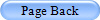## Practice ExamQuestion # 1 (Multiple Choice) Kinetic energy is a property only of the:

A) pressure
B) volume
C) area
D) temperature
E) density

Question # 2 (True/False) Pressure can be completely described in terms of gas molecule mass and average molecular velocities if one knows the number of gas molecules present.

A) True
B) False

Question # 3 (Multiple Choice) Temperatures held constant:

A) Boyles's law
C) both
D) neither

Question # 4 (Multiple Choice) The initial volume of nitrogen is 10 L at STP. If the temperature is raised to 512C and the pressure to 1520 mmHg, the new volume will be:

A) 0.01 L
B) 9.4 L
C) 8.7 L
D) 14.3 L
E) 13.2 L

Question # 5 (Multiple Choice) The volume of a gas, at constant pressure and number of molecules, varies in the following manner with respect to average molecular velocities

A) first power
B) second power
C) fourth power
D) eigth power

Question # 6 (Multiple Choice) Appropriate unit definition:

A) 760 atm = 1 mm Hg
B) 760 torr = 1 atm
C) both
D) neither

Question # 7 (True/False) -PAdx =-PdV, where P is pressure, A is area and dx where dx describes the distance moved by a piston and would be associated with compression of a gas in the piston.

A) True
B) False

Question # 8 (True/False) 1 Pa = 1 Newton/square meter

A) True
B) False

Question # 9 (Multiple Choice) P1V1 = P2V2

A) Charles' Law
B) Boyle's Law
C) Ideal Gas Law

Question # 10 (Multiple Choice) The number of molecules is held constant:

A) Combined gas law
B) Charles' Law
C) Boyle's Law
D) all of the above

Question # 11 (Multiple Choice) Appropriate definition: relationships between pressure, volume and area

A) pressure = volume/area
B) area = pressure/force
C) force = pressure x area
D) pressure = area/force

Question # 12 (Multiple Choice) Consider the equation P= dgh.

A) d refers to the downward pressure
B) g is the acceleration due to gravity
C) h is a constant of proportionality
D) p refers to viscosity

1 , 2 , 3 , 4 , 5 , 6 , 7 , 8 , 9 , 10 , 11 , 12
```

```

Question # 1 (Multiple Choice) Kinetic energy is a property only of the:

```

```

Question # 2 (True/False) Pressure can be completely described in terms of gas molecule mass and average molecular velocities if one knows the number of gas molecules present.

```

```

Question # 3 (Multiple Choice) Temperatures held constant:

```

```

Question # 4 (Multiple Choice) The initial volume of nitrogen is 10 L at STP. If the temperature is raised to 512C and the pressure to 1520 mmHg, the new volume will be:

```

```

Question # 5 (Multiple Choice) The volume of a gas, at constant pressure and number of molecules, varies in the following manner with respect to average molecular velocities

```

```

Question # 6 (Multiple Choice) Appropriate unit definition:

Answer: (B) 760 torr = 1 atm

```

```

Question # 7 (True/False) -PAdx =-PdV, where P is pressure, A is area and dx where dx describes the distance moved by a piston and would be associated with compression of a gas in the piston.

```

```

Question # 8 (True/False) 1 Pa = 1 Newton/square meter

```

```

Question # 9 (Multiple Choice) P1V1 = P2V2

```

```

Question # 10 (Multiple Choice) The number of molecules is held constant:

Answer: (D) all of the above

```

```

Question # 11 (Multiple Choice) Appropriate definition: relationships between pressure, volume and area

Answer: (C) force = pressure x area

```

```

Question # 12 (Multiple Choice) Consider the equation P= dgh.

Answer: (B) g is the acceleration due to gravity